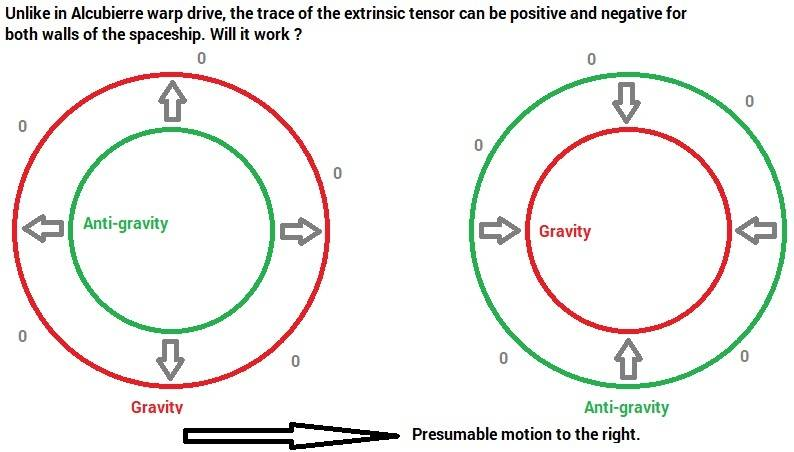# Energy distribution on Alcubierre walls

Hi, the trace of the extrinsic tensor K(Myu,Nu) in Alcubierre metric, relative to the foliation normal vector is non zero on the front x=rs+R and rear x=rs-R "walls". This is the normal volume component Tr =k(1,1)+k(2,2)+k(3,3) and should affect the Ricci tensor in Lorentzian/Minkowsky 4D. How do we know that the Weyl tensor is also zero out of the walls ? In order for the middle of the bubble to experience 0 g, all elements of the Riemann tensor have to be zero, is that correct ? In other words, does gravity vanish out of the walls or only Ricci tensor vanishes our of the walls ? If it is only the Ricci tensor then gravity is not close to zero at x=rs as claimed.

## Answers and Replies

Actually it is Tr = k(0,0)+k(1,1)+k(2,2)+k(4,4). Also (Tanh(x+R)-Tanh(x-R))/Tanh(Sig R) gets a weak maximum at x = 0. The simple model of anti-energy at the rear wall and energy at the front, does not yield this function and does not yield 0 g at the center of the spaceship. The pilot will be sucked towards the font wall if the rear was pure negative energy and the front was pure positive energy. Can anyone describe the energy and anti-energy distribution in the rear wall and in the font wall ?

Hi, the trace of the extrinsic tensor K(Myu,Nu) in Alcubierre metric, relative to the foliation normal vector is non zero on the rear x=rs+R (because it starts at negative rs) and front x=rs-R "walls". This is the normal volume component Tr =k(0,0)+k(1,1)+k(2,2)+k(3,3) and should affect the Ricci tensor in Lorentzian/Minkowsky 4D. How do we know that the Weyl tensor is also zero out of the walls ? In order for the middle of the bubble to experience 0 g, all elements of the Riemann tensor have to be zero, is that correct ? In other words, does gravity vanish out of the walls or only Ricci tensor vanishes our of the walls ? If it is only the Ricci tensor then gravity is not close to zero at x=rs as claimed. (Tanh((x+R)Sig)-Tanh((x-R)Sig))/Tanh(Sig R) gets a weak maximum at x = 0 for big Sig. The simple model of anti-energy at the rear wall and energy at the front, does not yield this function and does not yield 0 g at the center of the spaceship. The pilot will be sucked towards the front wall if the rear was pure negative energy and the front was pure positive energy. Can anyone describe the energy and anti-energy distribution in the rear wall and in the font wall ?

Last edited by a moderator:
Will that work despite the fact that (12) in Alcubierre's paper https://arxiv.org/pdf/gr-qc/0009013.pdf
is not fulfilled ? My intuition tells me yes and that the motion will be towards the left.berkeman
Mentor
Will that work despite the fact that (12) in Alcubierre's paper https://arxiv.org/pdf/gr-qc/0009013.pdf
is not fulfilled ? My intuition tells me yes and that the motion will be towards the left.Your image did not post. Try using the UPLOAD button to attach a PDF or JPEG image to your post.The following combination acts as a source and as a sink for, say, tiny material reference clocks that are scattered in the space-time of the spaceship which is illustrated by the image. Each wall is two hollowed balls of positive and negative energy. In the middle of the internal balls the gravity/anti-gravity is 0. Out of the external balls the gravity/anti-gravity is 0. It is not an Alcubierre compatible warp because Ricci scalar is not negative in one side and positive in the other. Will a warp still cause the spaceship to move to the right ?

#### Attachments

PeterDonis
Mentor
2020 Award
@Eytan Suchard where is all this coming from? Do you have a peer-reviewed reference? We would need to see a reference giving the actual math; your pictures and verbal descriptions are not sufficient.